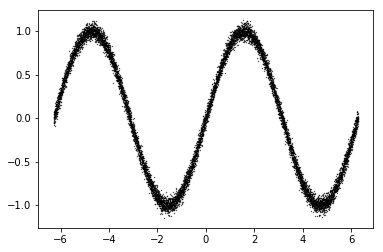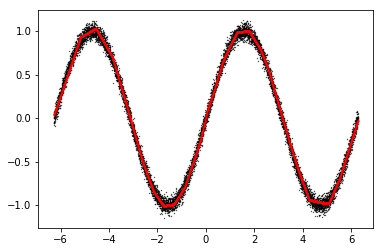0%

This tutorial will provide a brief overview of the core concepts and functionality of Tensorflow. This tutorial will cover the following:

1. What is Tensorflow
2. How to input data
3. How to perform computations
4. How to create variables
5. How to train a neural network for a simple regression problem
6. Tips and tricks

# 0. What is Tensorflow

Tensorflow is a framework to define a series of computations. You define inputs, what operations should be performed, and then Tensorflow will compute the outputs for you.

Below is a simple high-level example:

``````c = 3.0
``````

# 1. How to input data

Tensorflow has multiple ways for you to input data. One way is to have the inputs be constants:

``````c = 3.0
``````

However, having our inputs be constants is inflexible. We want to be able to change what data we input at runtime. We can do this using placeholders:

``````c0 = [3.]
c1 = [6.]
``````

But what if we don’t know the size of our input beforehand? One dimension of a tensor is allowed to be ‘None’, which means it can be variable sized:

``````Tensor("a_placeholder:0", shape=(?,), dtype=float32)
a shape: (?,)
Tensor("b_placeholder:0", shape=(?,), dtype=float32)
b shape: (?,)
c0 = [3.]
c1 = [3. 6.]
``````

# 2. How to perform computations

Now that we can input data, we want to perform useful computations on the data.

First, let’s create some data to work with:

``````a:
[[-1.]
[-2.]
[-3.]]
b:
[[1. 2. 3.]]
``````

We can do simple operations, such as addition:

``````b:
[[1. 2. 3.]]
c:
[[2. 4. 6.]]
``````

Be careful about the dimensions of the tensors, some operations may work even when you think they shouldn’t…

``````a:
[[-1.]
[-2.]
[-3.]]
b:
[[1. 2. 3.]]
c:
[[ 0.  1.  2.]
[-1.  0.  1.]
[-2. -1.  0.]]
``````

Also, some operations may be different than what you expect:

``````a:
[[-1.]
[-2.]
[-3.]]
b:
[[1. 2. 3.]]
c_elementwise:
[[-1. -2. -3.]
[-2. -4. -6.]
[-3. -6. -9.]]
c_matmul:
[[-14.]]
``````

Operations can be chained together:

``````b:
[[1. 2. 3.]]
c0:
[[2. 4. 6.]]
c1:
[[3. 5. 7.]]
``````

Finally, Tensorflow has many useful built-in operations:

``````b:
[[1. 2. 3.]]
c:
2.0
``````

# 3. How to create variables

Now that we can input data and perform computations, we want some of these operations to involve variables that are free parameters, and can be trained using an optimizer (e.g., gradient descent).

First, let’s create some data to work with:

``````b:
[[1. 2. 3.]]
``````

We’ll now create a variable

``````<tf.Variable 'myvar:0' shape=(1, 3) dtype=float32_ref>
``````

and check that it’s been added to Tensorflow’s variables list:

``````[<tf.Variable 'myvar:0' shape=(1, 3) dtype=float32_ref>]
``````

We can do operations with the variable just like any other tensor:

``````Tensor("Const:0", shape=(1, 3), dtype=float32)
<tf.Variable 'myvar:0' shape=(1, 3) dtype=float32_ref>
``````

Before we can run any of these operations, we must first initalize the variables

and then we can run the operations just as we normally would.

``````b:
[[1. 2. 3.]]
var:
[[2.0, 4.0, 6.0]]
c:
[[3. 6. 9.]]
``````

So far we haven’t said yet how to optimize these variables. We’ll cover that next in the context of an example.

# 4. How to train a neural network for a simple regression problem

We’ve discussed how to input data, perform operations, and create variables. We’ll now show how to combine all of these—with some minor additions—to train a neural network on a simple regression problem.

First, we’ll create data for a 1-dimensional regression problem:

``````<matplotlib.collections.PathCollection at 0x7ffa1bed23c8>
``````The below code creates the inputs, variables, neural network operations, mean-squared-error loss, gradient descent optimizer, and runs the optimizer using minibatches of the data.

``````0000 mse: 0.428
1000 mse: 0.067
2000 mse: 0.027
3000 mse: 0.016
4000 mse: 0.006
5000 mse: 0.002
6000 mse: 0.002
7000 mse: 0.001
8000 mse: 0.002
9000 mse: 0.001
``````

Now that the neural network is trained, we can use it to make predictions:

``````INFO:tensorflow:Restoring parameters from /tmp/model.ckpt

<matplotlib.collections.PathCollection at 0x7ff9dc62a550>
``````Not so hard after all! There is much more functionality to Tensorflow besides what we’ve covered, but you now know the basics.

# 5. Tips and tricks

##### (b) Check what variables have been created
``````I_am_a_variable:0
I_am_a_variable_too:0
``````
##### (c) Look at the tensorflow API, or open up a python terminal and investigate!
``````Help on function reduce_mean in module tensorflow.python.ops.math_ops:

reduce_mean(input_tensor, axis=None, keepdims=None, name=None, reduction_indices=None, keep_dims=None)
Computes the mean of elements across dimensions of a tensor. (deprecated arguments)

SOME ARGUMENTS ARE DEPRECATED. They will be removed in a future version.
Instructions for updating:
keep_dims is deprecated, use keepdims instead

Reduces `input_tensor` along the dimensions given in `axis`.
Unless `keepdims` is true, the rank of the tensor is reduced by 1 for each
entry in `axis`. If `keepdims` is true, the reduced dimensions
are retained with length 1.

If `axis` is None, all dimensions are reduced, and a
tensor with a single element is returned.

For example:

1234x = tf.constant([[1., 1.], [2., 2.]])tf.reduce_mean(x)  # 1.5tf.reduce_mean(x, 0)  # [1.5, 1.5]tf.reduce_mean(x, 1)  # [1.,  2.]

Args:
input_tensor: The tensor to reduce. Should have numeric type.
axis: The dimensions to reduce. If `None` (the default),
reduces all dimensions. Must be in the range
`[-rank(input_tensor), rank(input_tensor))`.
keepdims: If true, retains reduced dimensions with length 1.
name: A name for the operation (optional).
reduction_indices: The old (deprecated) name for axis.
keep_dims: Deprecated alias for `keepdims`.

Returns:
The reduced tensor.

@compatibility(numpy)
Equivalent to np.mean

Please note that `np.mean` has a `dtype` parameter that could be used to
specify the output type. By default this is `dtype=float64`. On the other
hand, `tf.reduce_mean` has an aggressive type inference from `input_tensor`,
for example:

1234x = tf.constant([1, 0, 1, 0])tf.reduce_mean(x)  # 0y = tf.constant([1., 0., 1., 0.])tf.reduce_mean(y)  # 0.5

@end_compatibility
``````
##### (d) Tensorflow has some built-in layers to simplify your code.
``````Help on function fully_connected in module tensorflow.contrib.layers.python.layers.layers:

fully_connected(inputs, num_outputs, activation_fn=<function relu at 0x7ffa20054c80>, normalizer_fn=None, normalizer_params=None, weights_initializer=<function variance_scaling_initializer.<locals>._initializer at 0x7ff9f2ecd158>, weights_regularizer=None, biases_initializer=<tensorflow.python.ops.init_ops.Zeros object at 0x7ff9f2ecc780>, biases_regularizer=None, reuse=None, variables_collections=None, outputs_collections=None, trainable=True, scope=None)

`fully_connected` creates a variable called `weights`, representing a fully
connected weight matrix, which is multiplied by the `inputs` to produce a
`Tensor` of hidden units. If a `normalizer_fn` is provided (such as
`batch_norm`), it is then applied. Otherwise, if `normalizer_fn` is
None and a `biases_initializer` is provided then a `biases` variable would be
created and added the hidden units. Finally, if `activation_fn` is not `None`,
it is applied to the hidden units as well.

Note: that if `inputs` have a rank greater than 2, then `inputs` is flattened
prior to the initial matrix multiply by `weights`.

Args:
inputs: A tensor of at least rank 2 and static value for the last dimension;
i.e. `[batch_size, depth]`, `[None, None, None, channels]`.
num_outputs: Integer or long, the number of output units in the layer.
activation_fn: Activation function. The default value is a ReLU function.
Explicitly set it to None to skip it and maintain a linear activation.
normalizer_fn: Normalization function to use instead of `biases`. If
`normalizer_fn` is provided then `biases_initializer` and
`biases_regularizer` are ignored and `biases` are not created nor added.
default set to None for no normalizer function
normalizer_params: Normalization function parameters.
weights_initializer: An initializer for the weights.
weights_regularizer: Optional regularizer for the weights.
biases_initializer: An initializer for the biases. If None skip biases.
biases_regularizer: Optional regularizer for the biases.
reuse: Whether or not the layer and its variables should be reused. To be
able to reuse the layer scope must be given.
variables_collections: Optional list of collections for all the variables or
a dictionary containing a different list of collections per variable.
outputs_collections: Collection to add the outputs.
trainable: If `True` also add variables to the graph collection
`GraphKeys.TRAINABLE_VARIABLES` (see tf.Variable).
scope: Optional scope for variable_scope.

Returns:
The tensor variable representing the result of the series of operations.

Raises:
ValueError: If x has rank less than 2 or if its last dimension is not set.
``````
##### (e) Use variable scope to keep your variables organized.
``````layer_0/W0:0
layer_0/b0:0
layer_1/W1:0
layer_1/b1:0
layer_2/W2:0
layer_2/b2:0
``````
##### (g) You can use tensorboard to visualize and monitor the training process.
If you like my blog, please donate for me.